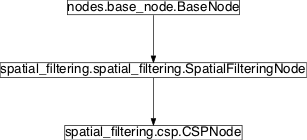# csp¶

## Module: `missions.nodes.spatial_filtering.csp`¶

Original and variants of the Common Spatial Pattern algorithm

The Common Spatial Pattern algorithm is a supervised method for spatial filtering. It is summarized e.g. in http://ieeexplore.ieee.org/xpls/abs_all.jsp?arnumber=4408441

Inheritance diagram for `pySPACE.missions.nodes.spatial_filtering.csp`:## `CSPNode`¶

class `pySPACE.missions.nodes.spatial_filtering.csp.``CSPNode`(retained_channels=None, relevant_indices=None, spatio_temporal=False, load_path=None, visualize_pattern=False, **kwargs)[source]

Common Spatial Pattern filtering

A node that implements the supervised common spatial pattern algorithm for spatial filtering as described in http://ieeexplore.ieee.org/xpls/abs_all.jsp?arnumber=4408441

The ordering of classes in this implementation is done in alphabetic order. This means when having the classes ‘Target’ and ‘Standard’, that the first channel is the best for ‘Standard’ and the last one the best for ‘Targets’, since the first one is the one with the highest eigenvalue.

To unify this spatial filter with other spatial filters for the final filter we arranged the channels in alternating order, meaning first, last, second, last but one, third, last but two, ... . This is done, such that the ordering of filters is according to importance of the filter, which is important, when deciding, which channels to choose. Nevertheless the channel names still correspond to the ordering of the eigenvalues.

Parameters
retained_channels:

Determines how many of the CSP pseudo channels are retained. Default is None which means “all channels”.

(optional, default: None)

relevant_indices:

Determines on which time indices the CSP filters are trained. Default is None which means “all time index”.

(optional, default: None)

spatio_temporal:

If this parameter is true, each sample is considered as a separate channel. This means that a time window with 64 channels and 25 observations is transformed into a time window with 64*25 channels with one observation. After filtering, the data is retransformed into a 64x25 time window.

(optional, default: False)

An absolute path from which the CSP patterns can be loaded. If not specified, these patterns are learned from the training data.

(optional, default: None)

visualize_pattern:

If value is true, a visualization of the learned CSP is stored. Therefore the parameter store needs to be set to true also.

(optional, default: False)

Exemplary Call

```-
node : CSP
parameters:
retained_channels : 32
relevant_indices : [-4,-3,-2,-1]
viszualize_pattern : True
store : True
```
Author: Created: Jan Hendrik Metzen (jhm@informatik.uni-bremen.de) 2008/12/09 CSP CSPNode TimeSeries

Class Components Summary

 `_execute`(data) Apply the learned spatial filters to the given data point. `_plot_spatial_values`(ax, spatial_values, ...) `_stop_training`([debug]) Finish the training, i.e. `_store_spatial_filter_plots`(filters, ...) Store spatial filter plots in the result_dir `_train`(data, label) Add the given data point along with its class label to the training set, i.e. `input_types` `is_supervised`() Returns whether this node requires supervised training `is_trainable`() Returns whether this node is trainable. `store_state`(result_dir[, index]) Stores this node in the given directory result_dir
`__init__`(retained_channels=None, relevant_indices=None, spatio_temporal=False, load_path=None, visualize_pattern=False, **kwargs)[source]
`is_trainable`()[source]

Returns whether this node is trainable.

`is_supervised`()[source]

Returns whether this node requires supervised training

`_train`(data, label)[source]

Add the given data point along with its class label to the training set, i.e. update the class’ covariance matrix

`_stop_training`(debug=False)[source]

Finish the training, i.e. solve the generalized eigenvalue problem Sigma_1*x = lambda*Sigma_2*x where Sigma_1 and Sigma_2 are the class covariance matrices and lambda and x are a eigenvalue, eigenvector pair.

`_execute`(data)[source]

Apply the learned spatial filters to the given data point.

`store_state`(result_dir, index=None)[source]

Stores this node in the given directory result_dir

static `_store_spatial_filter_plots`(filters, channel_names, result_dir)[source]

Store spatial filter plots in the result_dir

static `_plot_spatial_values`(ax, spatial_values, channel_names, title='')[source]
`input_types` = ['TimeSeries']